Reach Us+44-1522-440391
Classification of Canonical Bases for (<em>n-2</em>)-Dimensional Subspaces of n-Dimensional Vector Space | OMICS International
Journal of Generalized Lie Theory and Applications
All submissions of the EM system will be redirected to Online Manuscript Submission System. Authors are requested to submit articles directly to Online Manuscript Submission System of respective journal.

# Classification of Canonical Bases for (n-2)-Dimensional Subspaces of n-Dimensional Vector Space

Uladzimir Shtukar*

Associate Professor, Math/Physics Department, North Carolina Central University, Durham

*Corresponding Author:
Uladzimir Shtukar
Associate Professor, Math/Physics Department
North Carolina Central University
1801 Fayetteville Street, Durham
NC 27707, USA
Tel: 919-597-0375
E-mail: [email protected]

Received Date: August 25, 2016; Accepted Date: September 21, 2016; Published Date: September 30, 2016

Citation: Shtukar U (2016) Classification of Canonical Bases for (n−1)−dimensional Subspaces of n−Dimensional Vector Space. J Generalized Lie Theory Appl 10:241. doi:10.4172/1736-4337.1000241

Copyright: © 2016 Shtukar U. This is an open-access article distributed under the terms of the Creative Commons Attribution License, which permits unrestricted use, distribution, and reproduction in any medium, provided the original author and source are credited.

Visit for more related articles at Journal of Generalized Lie Theory and Applications

#### Abstract

Canonical bases for (n-1)-dimensional subspaces of n-dimensional vector space are introduced and classified in the article. This result is very prospective to utilize canonical bases at all applications. For example, maximal subalgebras of Lie algebras can be found using them.

#### Keywords

Vector space; Subspaces; Canonical bases

#### Introduction

The canonical bases for (n-1)-dimensional subspaces of n− dimensional vector space are introduced in the article, and all nonequivalent of them are classified (Theorem 2). This result generalizes a particular result for 5-dimensional subspaces of 6-dimensional vector space obtained in the previous article of the same author. To analyze the general case, reduced row echelon forms of matrices are utilized; about reduced row echelon forms . In addition to the principal result, all nonequivalent reduced row echelon forms for (n−1)×n matrices of the rank (n−1) are found and listed (Theorem 1).

Let V be an n− dimensional vector space with its standard basis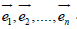. Let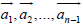be n − 1 linearly independent vectors in the space V where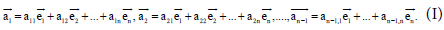The vectors (I) describe the possible bases for any V -dimensional subspace S of V.

Definition 1

Two bases are called equivalent if they generate the same subspace of V, and they are called nonequivalent if they generate two different subspaces of V .

We will associate the following (n−1) ×n matrix M with a basis (I)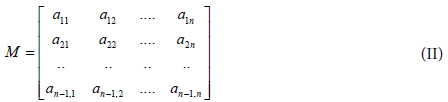Definition 2

Two matrices are called row equivalent (or just equivalent) if they have the same reduced row echelon form, and they are called nonequivalent if they have different reduced row echelon forms.

Definition 3

The basis (I) is called canonical if its vectors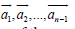are the corresponding rows in some reduced row echelon form of the matrix M.

Thus, there is one-to-one correspondence between nonequivalent canonical bases for (n−1)-dimensional subspaces of n−dimensional vector space and reduced row echelon forms for (n−1) ×n matrix M.

Remark

The standard linear operations with rows (vectors) will be utilized: (a) interchange any two rows, (b) multiply any row by a nonzero constant, (c) add a multiple of some row to another row .

Consider some examples with nonequivalent canonical bases for subspaces of small dimensional vector spaces.

Ex 1

Let V be 2-dimensional vector space with its standard basis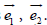Each 1-dimensional subspace S of V can be described as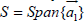where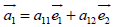. At least one component among a11, a12 of the vector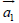is not zero. If a12≠0 then perform the operation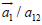, and we obtain the first canonical basis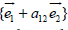. If a12=0 then perform the similar operation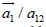,and we obtain the second canonical basis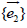. These two canonical bases are nonequivalent .

Ex 2

Let V be 3-dimensional vector space with its standard basis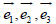. Consider any 2-dimensional subspace S of V that can be described as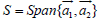where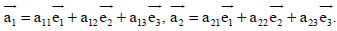This basis is equivalent to one and only one canonical basis from the next list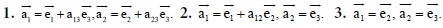Details of evaluation are omitted because it is easy. These last canonical bases generate the following matrices associated with them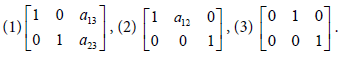Ex 3

Let V be 6-dimensional vector space with its standard basis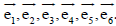Consider any 5-dimensional subspace S of V that can be described as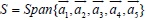where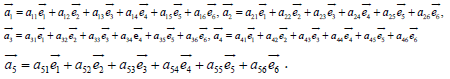These bases can be transformed into one and only one canonical basis from the next list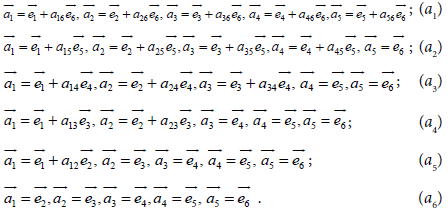All these canonical bases are nonequivalent. This result is obtained by the direct evaluation that generalizes Gauss-Jordan elimination method. The necessary details can be found in the different article of the same author .

The following matrices are associated with the last canonical bases (a1) – (a6):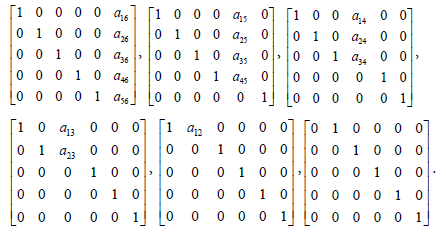The matrices obtained in Examples 2 and 3 are particular cases of the following matrices described by the next statement.

Theorem 1

All nonequivalent reduced row echelon forms of (n−1)×n matrices (n 3) of the rank (n−1) are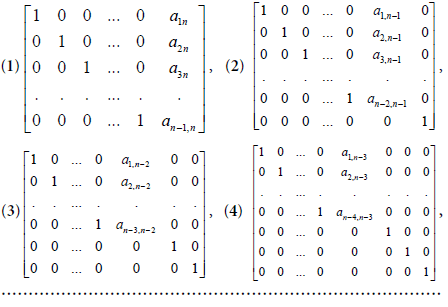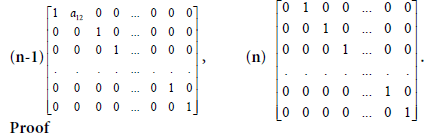We use the mathematical induction method. The statement is true for n=3 and n=6 according Examples 2 and 3.

Suppose that the statement is true for some dimension n, and prove it for the next dimension n + 3. For it, consider the following matrix of the size n ×(n+1)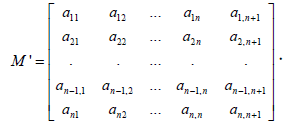Go to the (n−1) ×n submatrix located in the left upper corner of M′. According the assumption, this submatrix can be transformed into one and only one reduced row echelon form described in the cases (1), (2), (3), (4), ….(n−1), (n). This means that we can replace the mentioned submatrix by one of the given reduced forms, and analyze the new matrix. We will analyze and show all details in steps 1, 2, 3, and steps (n-1), (n). All other steps are very similar to the steps 1, 2, 3, therefore they are omitted.

Step 1

Substitute the left upper (n−1) ×n submatrix in M by the matrix (1). We have the following matrix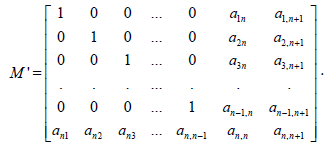Perform the following operations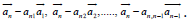. We obtain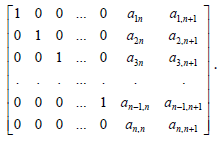Consider the elements an,n, an, n+1 in the last matrix. At least one of them is not zero because the rank of the matrix M′ is equal n. If an,n ≠ 0 then perform the operation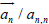first, and the operations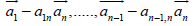after the first one. We obtained the matrix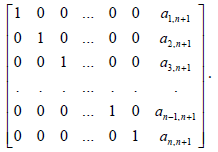This is the matrix of the type (1) as it’s needed. If an, n = 0 then an,n+1 ≠ 0. Perform the operation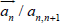first, and the operations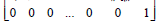We obtain the matrix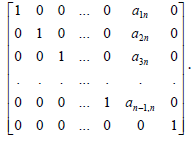This is the matrix of the type (2) as it’s needed. Step 1 is done.

Step 2

Substitute the left upper (n−1) ×n submatrix in M by the matrix (2). We have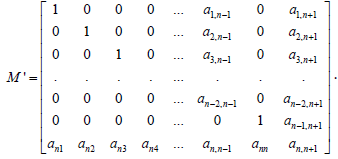Perform the operations,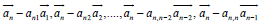. We obtain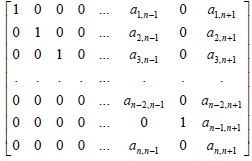At least one element among an, n1, an, n+1 is not zero. If an, n1≠0 then perform the operation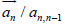first, and the operations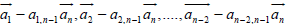after the first operation. We obtain the following matrix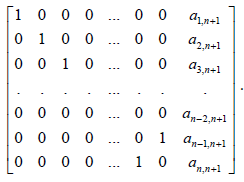The last matrix is row equivalent to the matrix (1) as it’s needed. If an, n−1=0 then an, n+1≠ 0. Perform the operation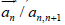first, and the operations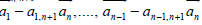after the first one. We obtain the following matrix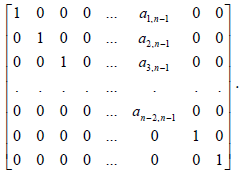The last matrix is a matrix of the type (3) as it’s needed.

Step 3

Substitute the left upper (n−1) ×n submatrix in M by the matrix (3). We have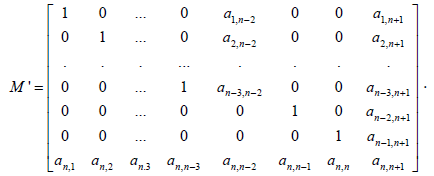Perform the operations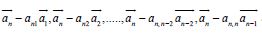. We obtain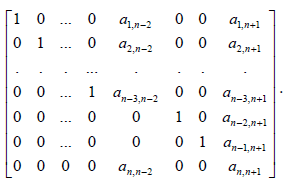At least one element among an, n2, an, n+1 is not zero in this matrix. If an, n2 then perform the operation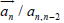first, and the operations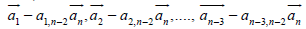after the first operation. We obtain the following matrix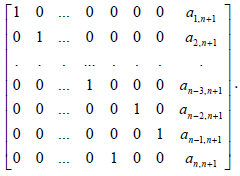The last matrix is row equivalent to the matrix (1) as it’s needed. If an, n−2= 0 then an, an,n+1≠0. Perform the operation,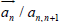first, and the operations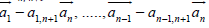after the first one. We obtain the following matrix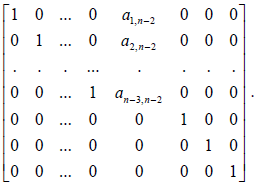We have the matrix that is equivalent to the matrix of the type (4) as it’s needed.

Step (n−1). Substitute the left upper (n−1) ×n submatrix in M′ by the matrix (n−1). We have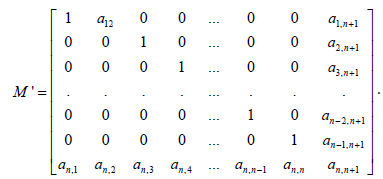Perform the operations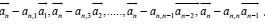. We obtain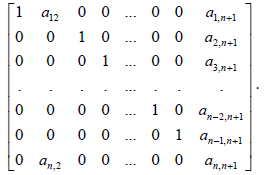At least one element among an, 2, an, n+1 is not zero in this matrix. If an, 2≠0 then perform the operation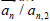first, and the operation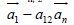after the first operation. We obtain the following matrix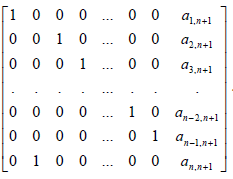If interchange rows in the last matrix, we obtain the matrix (1) as it’s needed. If an, 2=0 then an, n+1≠0. Perform the operation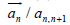first, and the operations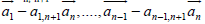after the first one. We obtain the following matrix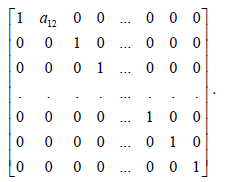It is the matrix of the type (n-1) as we need.

Step n

Substitute the left upper (n−1) ×n submatrix in M by the matrix (n). We have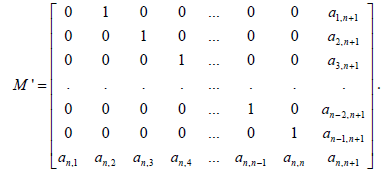Perform the operations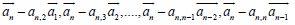We obtain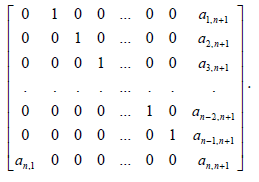At least one element among an, 1, an, n+1 is not zero in this matrix. If an, 1≠0 then perform the operation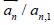and interchange the rows. We obtain the matrix of the type (1) as it’s needed. If an, 1=0 then an, n+10. Perform the operation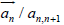first, and the operations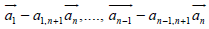after the first one. We obviously obtain the matrix of the type (n) as it’s needed. The proof is done.

Theorem 1 can be transformed into the next statement that describes canonical bases for (n−1) −dimensional subspaces of n− dimensional vector space.

Theorem 2

All nonequivalent canonical bases for (n−1) −dimensional subspaces of n−dimensional vector space (if (n≥2)) are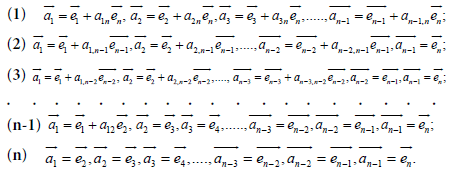The statement is true for n≥3 because of Theorem 1. The additional case n = 2 is included in Theorem 2 because of Example 1.

#### Final Remark

The canonical bases introduced in the article are a powerful instrument that can be utilized in all applications of Linear Algebra. For example, all maximal subalgebras of any Lie algebra can be found using canonical bases listed in Theorem 2.

#### References

Select your language of interest to view the total content in your interested language

### Article Usage

• Total views: 9382
• [From(publication date):
December-2016 - Aug 18, 2019]
• Breakdown by view type
• HTML page views : 9254
• PDF downloads : 128

## Post your commentCan't read the image? click here to refresh
###### Peer Reviewed Journals

Make the best use of Scientific Research and information from our 700 + peer reviewed, Open Access Journals

International Conferences 2019-20

Meet Inspiring Speakers and Experts at our 3000+ Global Annual Meetings

Top